﻿

### On some arithmetic properties of nite groups

#### Abstract

Let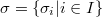$\sigma =\{\sigma_{i} | i\in I\}$ be some partition of the set of all primes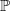$\Bbb{P}$. A group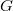$G$ is called \emph{$\sigma$-primary} if$G$ is a finite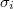$\sigma_{i}$-group for some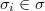$\sigma_{i}\in \sigma$. We say that a finite group$G$ is: \emph{$\sigma$-soluble} if every chief factor of$G$ is$\sigma$-primary; \emph{$\sigma$-nilpotent} if$G$ is the direct product of some$\sigma$-primary groupsBeing based on these concepts, we develop and unify some aspects of the theories of soluble, nilpotent, supersoluble and quasinilpotent groups, the subgroup lattices theory, the theories of generalized quasinormal and generalized subnormal subgroups

DOI Code: 10.1285/i15900932v36suppl1p65

Keywords: finite group; Hall subgroup; $\Pi$-full group; $\Pi$-subnormal subgroup; $\sigma$-soluble group; $\sigma$-nilpotent subgroup

Full Text: PDF